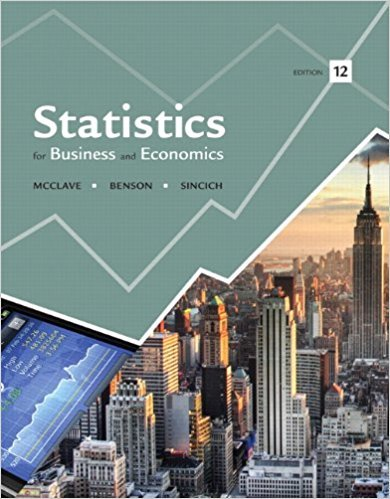×
Get Full Access to Statistics For Business And Economics - 12 Edition - Chapter 9 - Problem 64e
Get Full Access to Statistics For Business And Economics - 12 Edition - Chapter 9 - Problem 64e

×

# Suppose you conduct a 4 X 3 factorial experiment.a. HowISBN: 9780321826237 51

## Solution for problem 64E Chapter 9

Statistics for Business and Economics | 12th Edition

• Textbook Solutions
• 2901 Step-by-step solutions solved by professors and subject experts
• Get 24/7 help from StudySoup virtual teaching assistantsStatistics for Business and Economics | 12th Edition

4 5 1 412 Reviews
14
5
Problem 64E

Problem 64E

Suppose you conduct a 4 X 3 factorial experiment.

a. How many factors are used in the experiment?

b. Can you determine the factor type(s)—qualitative or quantitative—from the information given? Explain.

c. Can you determine the number of levels used for each factor? Explain.

d. Describe a treatment for this experiment and determine the number of treatments used.

e. What problem is caused by using a single replicate of this experiment? How is the problem solved?

Step-by-Step Solution:
Step 1 of 3

STAT 2004 CHAPTER ONE: INTRODUCTION TO DATA POPULATIONS AND SAMPLES  Population- Represents all people or things of interest.  Sample- Observed/measured subset of a population.  Data- Observations that form the backbone of a statistical investigation.  Summary statistic- A single number that summarizes a large amount of data.  Variables- Measured or observed characteristics of data. o Categorical variable- Responses themselves are categories.  Nominal- Unordered levels.  Ordinal- Ordered levels. o Numerical variable- Counts/measures information. Can take a wide range of numerical values. Sensible to add, subt

Step 2 of 3

Step 3 of 3

##### ISBN: 9780321826237

Unlock Textbook Solution